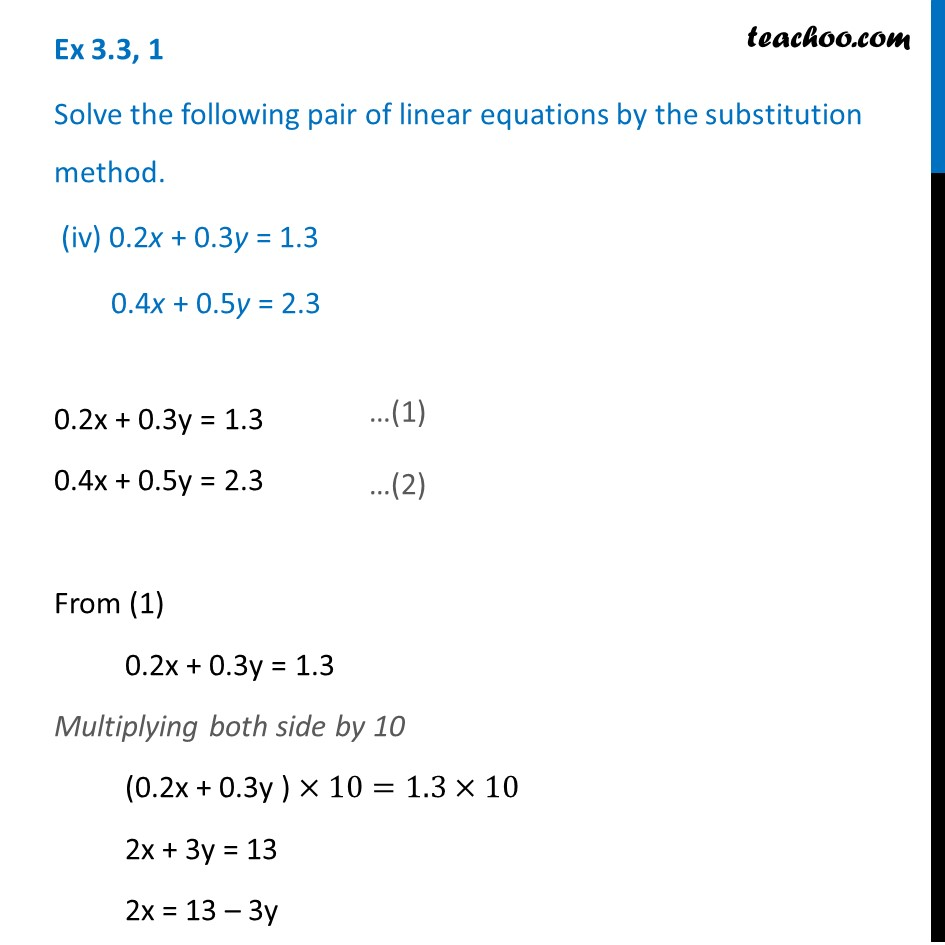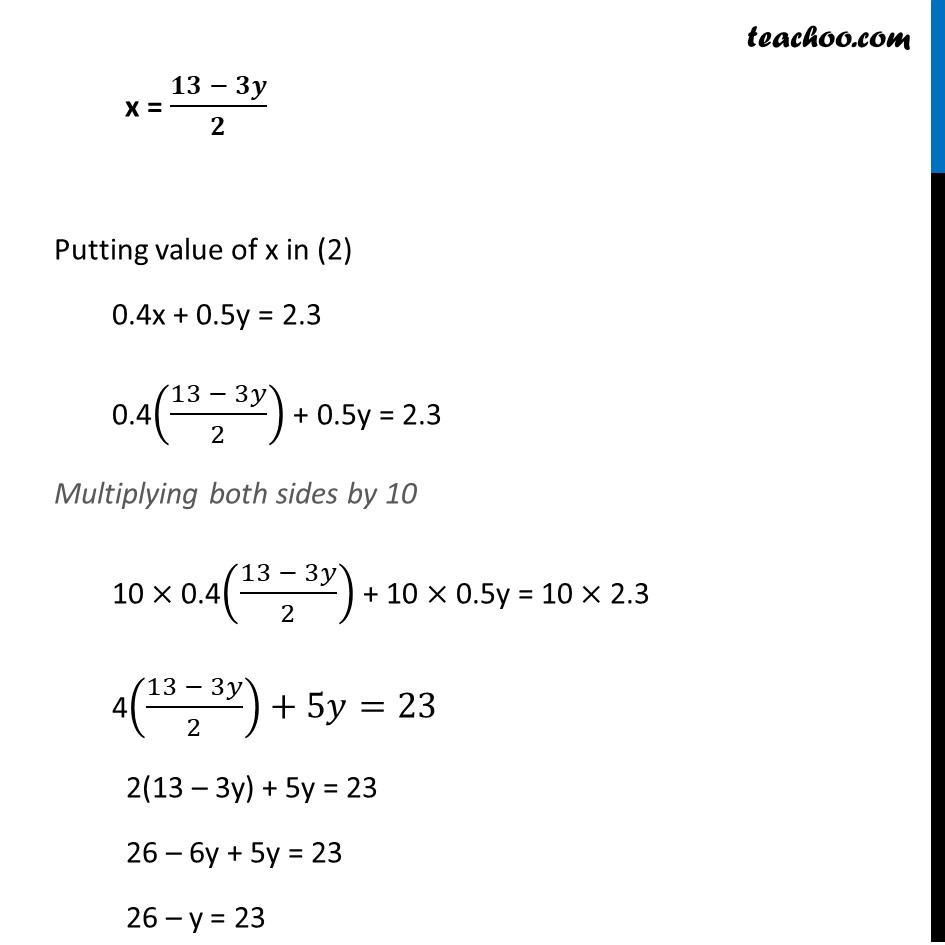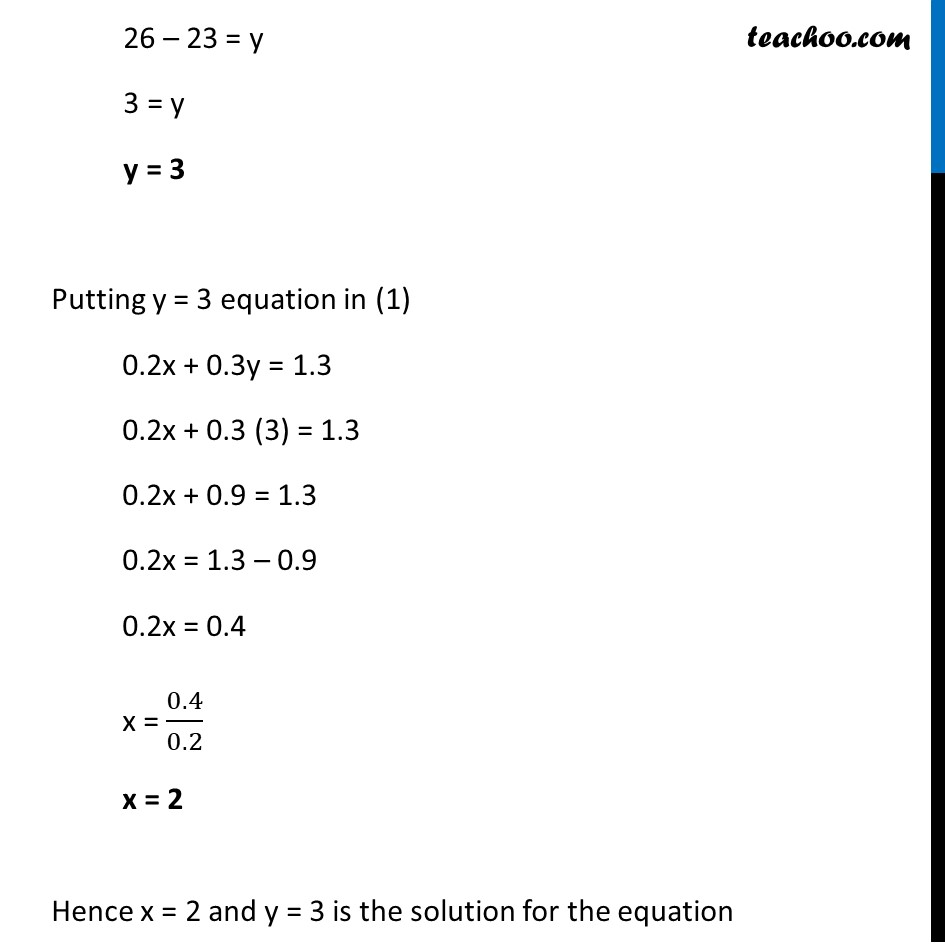Ex 3.3

Chapter 3 Class 10 Pair of Linear Equations in Two Variables
Serial order wiseGet live Maths 1-on-1 Classs - Class 6 to 12

### Transcript

Ex 3.3, 1 Solve the following pair of linear equations by the substitution method. (iv) 0.2x + 0.3y = 1.3 0.4x + 0.5y = 2.3 0.2x + 0.3y = 1.3 0.4x + 0.5y = 2.3 From (1) 0.2x + 0.3y = 1.3 Multiplying both side by 10 (0.2x + 0.3y ) ×10=1.3×10 2x + 3y = 13 2x = 13 – 3y x = (𝟏𝟑 − 𝟑𝒚)/𝟐 Putting value of x in (2) 0.4x + 0.5y = 2.3 0.4((13 − 3𝑦)/2) + 0.5y = 2.3 Multiplying both sides by 10 10 × 0.4((13 − 3𝑦)/2) + 10 × 0.5y = 10 × 2.3 4((13 − 3𝑦)/2)+5𝑦=23 2(13 – 3y) + 5y = 23 26 – 6y + 5y = 23 26 – y = 23 26 – 23 = y 3 = y y = 3 Putting y = 3 equation in (1) 0.2x + 0.3y = 1.3 0.2x + 0.3 (3) = 1.3 0.2x + 0.9 = 1.3 0.2x = 1.3 – 0.9 0.2x = 0.4 x = 0.4/0.2 x = 2 Hence x = 2 and y = 3 is the solution for the equation